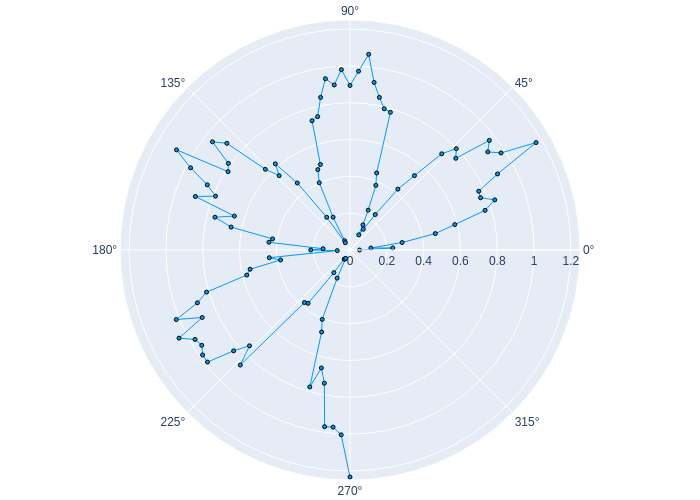# Polar Plotsusing Plots
plotlyjs()
Θ = range(0, stop = 1.5π, length = 100)
r = abs.(0.1 * randn(100) + sin.(3Θ))
plot(Θ, r, proj = :polar, m = 2)